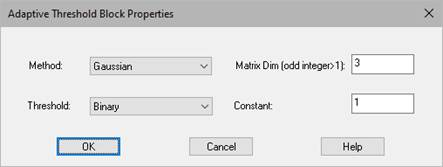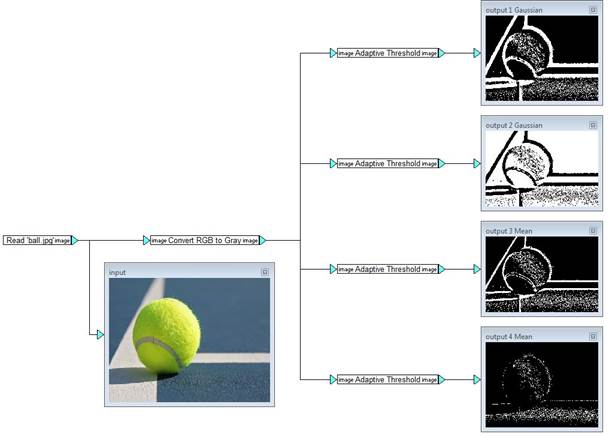Block Category: Operations

Input Image Requirements: Gray scale

Description: The Adaptive Threshold block creates a binary image by classifying each pixel as either dark or light based on whether its intensity value is above or below the specified threshold.Constant: Indicates a constant value subtracted from the mean or weighted mean. Specify the constant as an integer.

Matrix Dim: Indicates the matrix size of the pixel neighborhood used to calculate a threshold value for a pixel. Specify the size as an odd integer greater than 1.

#### Method

Gaussian: Indicates that the threshold value T(x,y) is a weighted sum (cross-correlation with a Gaussian window) of the pixel neighborhood of (x,y) minus the specified constant.

Mean: Indicates that the threshold value T(x,y) is the mean of the pixel neighborhood of (x,y) minus the specified constant.

Threshold: Indicates the thresholding type. There are two choices: Binary and Binary Invert.

#### Example

In this example, four binary images are created by varying the algorithm, threshold, size, and constant values. In all but Output1 (bottom display), the mean of neighborhood size of 35 x 35 is applied to the adaptive threshold. In Output1, a smaller neighborhood size is used and results in a blurrier image. Output 2 uses the Binary (rather than Binary Invert) threshold type.